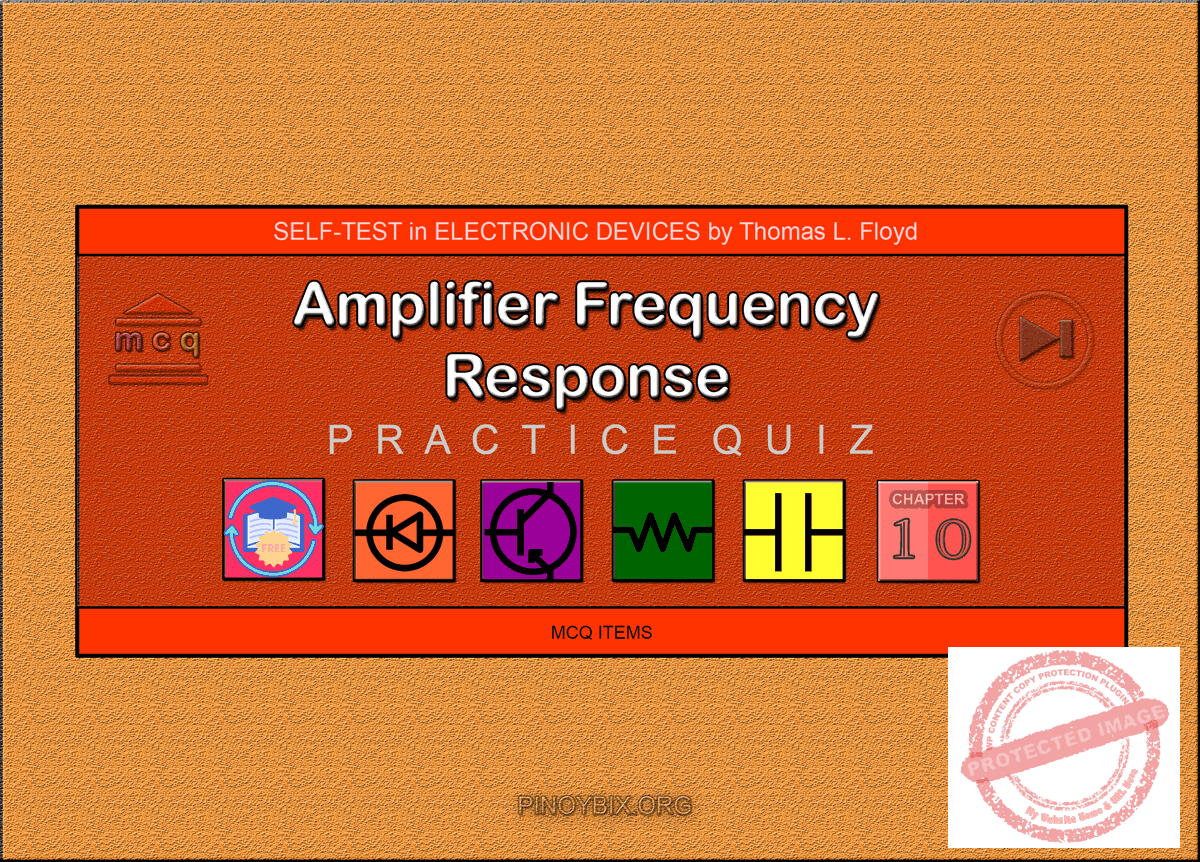# Floyd Self-test in Amplifier Frequency Response

(Last Updated On: December 5, 2019)This is the Self-test in Chapter 10: Amplifier Frequency Response from the book Electronic Devices Conventional Current Version, 9th edition by Thomas L. Floyd. If you are looking for a reviewer in Electronics Engineering this will definitely help you before taking the Board Exam.

#### Floyd Self-test Chapter 10 Topic Outline

• Floyd Self-test in Basic Concepts of Amplifier Frequency Response
• Floyd Self-test in The Decibel
• Floyd Self-test in Low-Frequency Amplifier Response
• Floyd Self-test in High-Frequency Amplifier Response
• Floyd Self-test in Total Amplifier Frequency Response
• Floyd Self-test in Frequency Response of Multistage Amplifiers
• Floyd Self-test in Frequency Response Measurements

If you are looking for the Multiple Choice Questions in Floyd’s Electronic Devices proceed to

#### Start Practice Exam Test Questions

Choose the letter of the best answer in each questions.

1. The low-frequency response of an amplifier is determined in part by

(a) the voltage gain

(b) the type of transistor

(c) the supply voltage

(d) the coupling capacitors

Solution:

2. The high-frequency response of an amplifier is determined in part by

(a) the gain-bandwidth product

(b) the bypass capacitor

(c) the internal transistor capacitances

(d) the roll-off

Solution:

3. The Miller input capacitance of an amplifier is dependent, in part, on

(a) the input coupling capacitor

(b) the voltage gain

(c) the bypass capacitor

(d) none of these

Solution:

4. The decibel is used to express

(a) power gain

(b) voltage gain

(c) attenuation

(d) all of these

Solution:

5. When the voltage gain is 70.7% of its midrange value, it is said to be

(a) attenuated

(b) down 6 dB

(c) down 3 dB

(d) down 1 dB

Solution:

6. In an amplifier, the gain that occurs between the lower and upper critical frequencies is called the

(a) critical gain

(b) midrange gain

(c) bandwidth gain

(d) decibel gain

Solution:

7. A certain amplifier has a voltage gain of 100 at midrange. If the gain decreases by 6 dB, it is equal to

(a) 50

(b) 70.7

(c) 0

(d) 20

Solution:

8. The gain of a certain amplifier decreases by 6 dB when the frequency is reduced from 1 kHz to 10 Hz. The roll-off is

(c) -3dB/octave

(d) -6dB/octave

Solution:

9. The gain of a particular amplifier at a given frequency decreases by 6 dB when the frequency is doubled. The roll-off is

(c) -6dB/octave

Solution:

10. The lower critical frequency of a direct-coupled amplifier with no bypass capacitor is

(a) variable

(b) 0 Hz

(c) dependent on the bias

(d) none of these

Solution:

11. At the upper critical frequency, the peak output voltage of a certain amplifier is 10 V. The peak voltage in the midrange of the amplifier is

(a) 7.07 V

(b) 6.37 V

(c) 14.14 V

(d) 10 V

Solution:

12. The high-frequency response of an amplifier is determined by the

(a) coupling capacitors

(b) bias circuit

(c) transistor capacitances

(d) all of these

Solution:

13. The Miller input and output capacitances for a BJT inverting amplifier depend on

(a) Cbc

(b) βac

(c) Av

Solution:

14. The bandwidth of an amplifier is determined by

(a) the midrange gain

(b) the critical frequencies

(c) the roll-off rate

(d) the input capacitance

Solution:

15. An amplifier has the following critical frequencies: 1.2 kHz, 950 Hz, 8 kHz, and 8.5 kHz. The bandwidth is

(a) 7550 Hz

(b) 7300 Hz

(c) 6800 Hz

(d) 7050 Hz

Solution:

16. Ideally, the midrange gain of an amplifier

(a) increases with frequency

(b) decreases with frequency

(c) remains constant with frequency

(d) depends on the coupling capacitors

Solution:

17. The frequency at which an amplifier’s gain is 1 is called the

(a) unity-gain frequency

(b) midrange frequency

(c) corner frequency

(d) break frequency

Solution:

18. When the voltage gain of an amplifier is increased, the bandwidth

(a) is not affected

(b) increases

(c) decreases

(d) becomes distorted

Solution:

19. If the fT of the transistor used in a certain amplifier is 75 MHz and the bandwidth is 10 MHz, the voltage gain must be

(a) 750

(b) 7.5

(c) 10

(d) 1

Solution:

20. In the midrange of an amplifier’s bandwidth, the peak output voltage is 6 V. At the lower critical frequency, the peak output voltage is

(a) 3 V

(b) 3.82 V

(c) 8.48 V

(d) 4.24 V

Solution:

21. The dominant lower critical frequency of a multistage amplifier is the

(a) lowest fcl

(b) highest fcl

(c) average of all the fcl’s

(d) none of these

Solution:

22. When the critical frequencies of all of the stages are the same, the dominant critical frequency is

(a) higher than any individual fcl

(b) lower than any individual fcl

(c) equal to the individual fcl’s

(d) the sum of all individual fcl’s

Solution:

23. In the step response of a noninverting amplifier, a longer rise time means

(a) a narrower bandwidth

(b) a lower fcl

(c) a higher fcu

Solution:

### Complete List of Floyd Self-test in Electronic Devices

Show Simple Act of Caring!

Please consider subscribing to our YouTube Channel for engineering video tutorials. You can also find us on Twitter and Facebook. Lastly, Our app is now available on Google Play, Pinoybix Elex

© 2014 PinoyBIX Engineering. © 2019 All Rights Reserved | How to Donate? |#### Pre-board in Electronics Engineering

Pre-board in Electronics Engineering - Answers

#### Pre-board in Communications Engineering

Pre-board in Communications Engineering - Answers

#### GEAS Solution

Dynamics problem Economics problem Physics problem Statics problem Strength problem Thermodynamics problem

#### Questions and Answers in GEAS

Engineering Economics Engineering Laws and Ethics Engineering Management Engineering Materials Engineering Mechanics General Chemistry Physics Strength of Materials Thermodynamics
Consider Simple Act of Caring!: LIKE MY FB PAGE

Our app is now available on Google Play, Pinoybix Elex